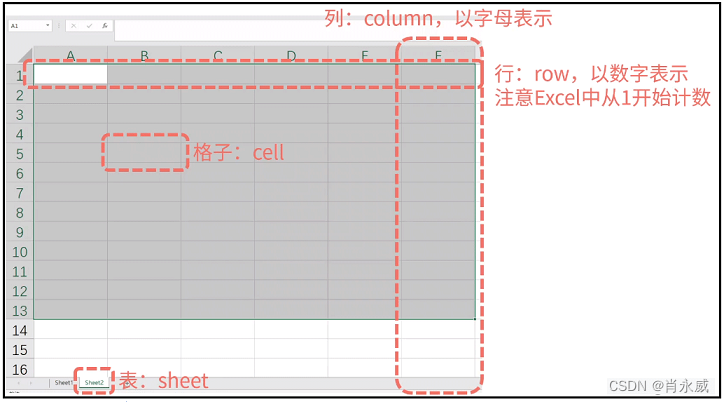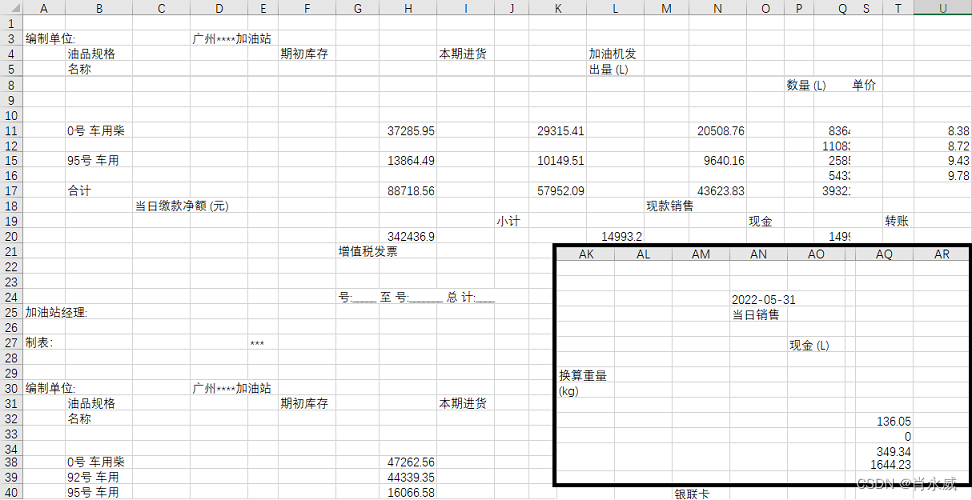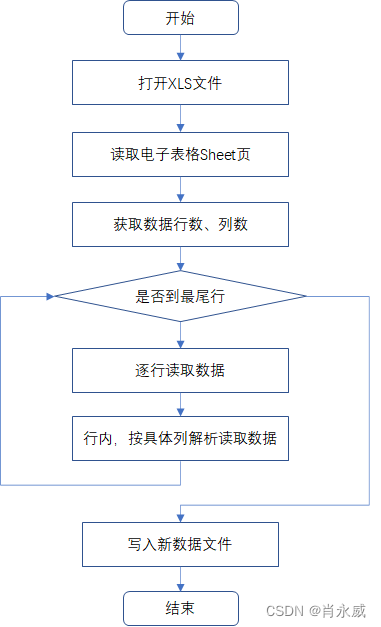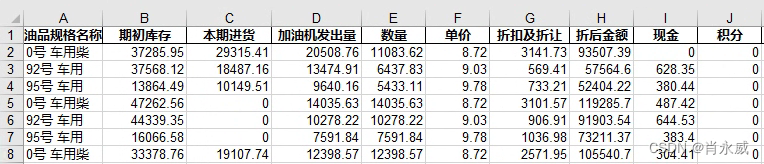# Python读取电子表格方法

• 一个电子表格文档（Excel）称为一个工作簿
• 一个工作簿保存在一个扩展名为XLS（.xlsx）的文件中
• 一个工作簿可以包含多个表（sheet）
• 在特定行和列的方格称为单元格、格子

• XLS是excel2003及以前版本所生成的文件格式
• XLSX是excel2007及以后版本所生成的文件格式Python对excel文件的读写功能的模块有以下三种：

• xlwt：对 xls 格式的 Excel 文件进行写入；
• xlrd：对 xls 格式的 Excel 文件进行读取；
• OpenpPyXL 实现了对 xlsm 、xlsx 开放电子表格格式的读写。

``````pip install -i https://pypi.tuna.tsinghua.edu.cn/simple xlrd
pip install -i https://pypi.tuna.tsinghua.edu.cn/simple xlwt
``````

# 原始数据``````data_dict = {
'油品规格名称':{'colname':'oilname','id':1},
'期初库存':{'colname':'openinginventory','id':7},
'本期进货':{'colname':'currentpurchase','id':10},
'加油机发出量':{'colname':'sendout','id':13},
'数量':{'colname':'values','id':16},
......
``````

# 数据解析过程框图# 实践代码示例

• 打开XLS工作薄：
workbook = xlrd.open_workbook(filename)

• 三种方式获取工作表
table = workbook.sheet_by_index(0)，按索引顺序
table = workbook.sheets()
table = workbook.sheet_by_name(‘日报表202110-202210’)，按sheet名称

• 读取单元格数据：
value = table.cell_value(rowx=2, colx=0)，rowx，colx分别是行、列索引数（注意：从0开始）

``````import xlrd

# filename是文件的路径名称，如果路径或者文件名有中文给前面加一个r拜师原生字符。
workbook = xlrd.open_workbook(filename=r'日报表202110-202210.XLS')
#workbook = xlrd.open_workbook(filename=r'2日报表202110-202210.XLS',encoding_override='utf-8')

# 获取第一个sheet表格
table = workbook.sheets()
# 初始化，按行定义list
dat_row = []
# 数据单元格，列的索引位置
cols_index = [1,7,10,13,16,20,23,30,...,83,87]
# 批发油的情况，价格不一致（猜测）
cols_index_0 = [16,20,23,30,...]
cols_len = len(cols_index)
cols_len_0 = len(cols_index_0)

# 获取sheet中有效行数
rows = table.nrows
# 表头与首行数据间隔函数
irow_bank = 5
irow = 0
icol = cols_index
while irow < rows:
if table.cell_value(rowx=irow, colx=0) == '编制单位:':
cell_vd = table.cell_value(rowx=irow, colx=39)   # 日报时间索引位置39

cell_v = table.cell_value(rowx=irow, colx=icol)

if cell_v == '名称':
#irow = irow + irow_bank
# 跨过空行
irow = irow + 1

while len(table.cell_value(rowx=irow, colx=icol)) == 0:
irow = irow + 1

while table.cell_value(rowx=irow, colx=icol) != '合计':
dat_col = []
for j in range(cols_len):
dat_col.append(table.cell_value(rowx=irow, colx=cols_index[j]))
# 批发油的情况，价格不一致
if len(table.cell_value(rowx=irow+1, colx=icol)) == 0:
irow = irow + 1
for j in range(cols_len_0):
dat_col.insert(4+j,table.cell_value(rowx=irow, colx=cols_index_0[j]))
else:
for j in range(cols_len_0):
dat_col.append(0)

dat_col.append(cell_vd)
print(dat_col)
dat_row.append(dat_col)
irow = irow + 1

# 逐行扫描
irow = irow + 1

irow

``````

``````import pandas as pd

df.to_excel('dd.xls',encoding='utf_8_sig',index=False)
``````# 总结

Python按单元格读取复杂电子表格（Excel）数据技术上比较成熟，易操作。需要注意事项：

• 由于数据文件来自第三方，可能存在编码问题（中文乱码），最好拿到手后，在文件处理的系统上，再另存新文件（本次工作，就是遇到类似问题，花费变天时间也没有解决编码转换或者加密问题，简单的另存解决。
• 数据处理过程，还是使用pandas更加专业。

lainwith. python实现——处理Excel表格（超详细）. CSDN博客. 2021.10